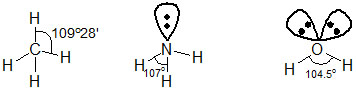#### Consider the molecules CH4, NH3 and H2O. Which of the given statements is false? Option 1) The H - C - H bond angle in CH4, the H - N - H bond angle in NH3, and the H - O - H bond angle in H2O are all greater than 90o. Option 2) The H - O - H bond angle in H2O is larger than the H - C - H bond angle in CH4. Option 3) The H - O - H bond angle in H2O is smaller than the H - N - H bond angle in NH3. Option 4) The H - C - H bond angle in CH4 is larger than the H - N - H bond angle in NH3.As we learnt in

VSEPR Theory -

1.  The shape of the molecule is determined by repulsions between all of the electron pair present in valence shell.

2.  Order of repulsion3  Repulsion among the bond pair is directly proportional to the bond  order and electronegativity difference between the central atom and the other atom.

-From the above fugure it is clear that H—O—H bond angle inis smaller than H—C—H bond angles in.

Option 1)

The H - C - H bond angle in CH4, the H - N - H bond angle in NH3, and the H - O - H bond angle in H2O are all greater than 90o.

This option is incorrect

Option 2)

The H - O - H bond angle in H2O is larger than the H - C - H bond angle in CH4.

This option is correct

Option 3)

The H - O - H bond angle in H2O is smaller than the H - N - H bond angle in NH3.

This option is incorrect

Option 4)

The H - C - H bond angle in CH4 is larger than the H - N - H bond angle in NH3.

This option is incorrect

##### Posted by